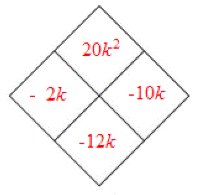### Home > CCA > Chapter 8 > Lesson 8.1.3 > Problem8-31

8-31.

Factor the following quadratic expressions, if possible.

1.  $k^2-12k+20$

Use a generic rectangle and a diamond to solve.Generic rectangle left edge top$-2$ interior top left$-2k$ interior top right$20$ left edge bottom$k$ interior bottom left$k^2$ interior bottom right$-10k$ bottom edge left$k$ bottom edge right$10$
1.  $6x^2+17x−14$

$(2x+7)(3x−2)$

1.  $x^2−8x+16$

1.  $9m^2−1$

Insert a $0m$ between the $9m^2$ and the $−1$.

1. Parts (a) through (c) are trinomials while part (d) is a binomial, yet they are all quadratics. What makes each of them a quadratic?

• What the highest power of the variable in each of the problems?